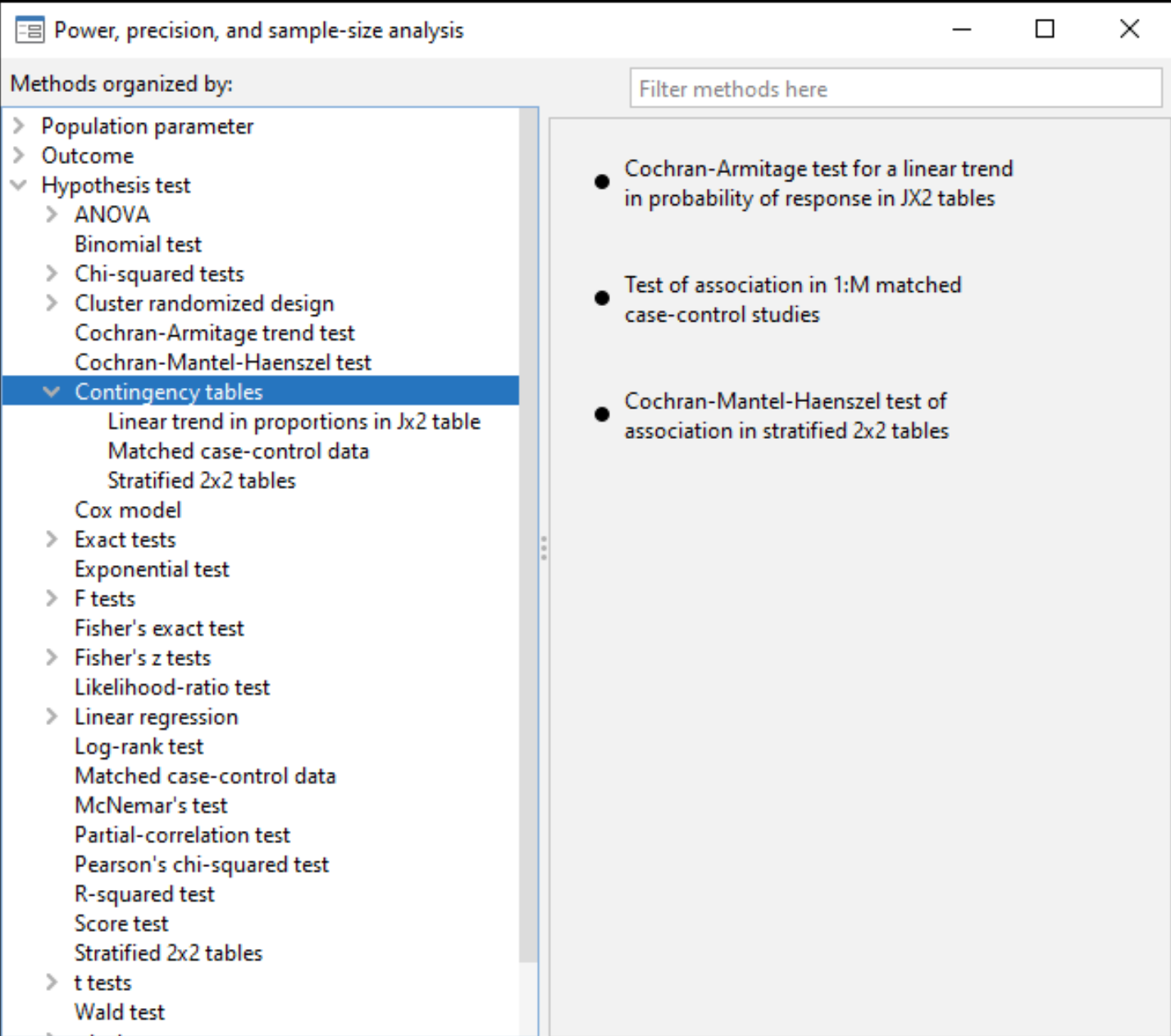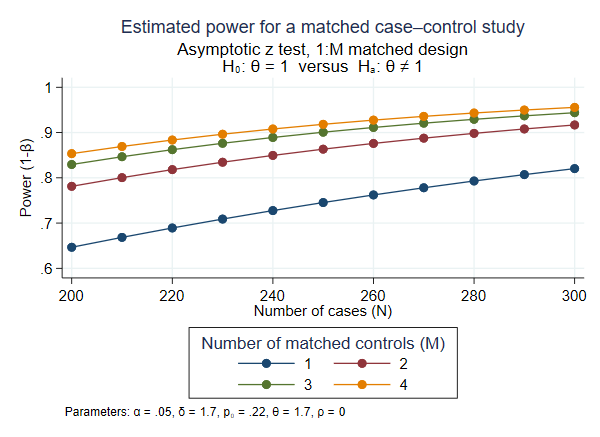»  Home »  Products »  Features »  Power analysis for contingency tables

## Power analysis for contingency tables

Stata's power performs various power and sample-size analysis. Among these, there are three methods for contingency tables.

You can compute power, sample size, and effect size. Enter any two and get the third. You can specify single values or, to compare multiple scenarios, ranges of values of study parameters. You can obtain results either in tabular form or as a graph.

Also, do not forget the facilities to easily add your own new methods to power.

### Contingency tables

Stata's power provides three methods for contingency-tables analysis. Go to the menu Statistics -> Power, precision, and sample size and select Contingency tables under Hypothesis test for point-and-click analysis. You can also work interactively.power cmh performs power and sample-size analysis for a Cochran–Mantel–Haenszel test of association in stratified 2x2 tables. It computes sample size, power, or effect size (common odds ratio) given other study parameters. It provides computations for designs with unbalanced stratum sizes as well as unbalanced group sizes within each stratum.

power mcc performs power and sample-size analysis for a test of association between a risk factor and a disease in 1:M matched case–control studies. It computes sample size, power, or effect size (odds ratio) given other study parameters.

power trend performs power and sample-size analysis for a Cochran–Armitage test of a linear trend in a probability of response in Jx2 tables. The rows of the table correspond to ordinal exposure levels. The command computes sample size or power given other study parameters. It provides computations for unbalanced designs and for unequally spaced exposure levels (doses). With equally spaced exposure levels, a continuity correction is available.

Here we use power mcc to demonstrate power and sample-size analysis for a matched case–control study. Consider a study comparing the odds of developing lung cancer among smokers and nonsmokers. How many case–control pairs do we need to achieve 80% power of detecting an odds ratio of 1.7 with a 5% significance if we use a two-sided association test? From previous studies, the probability of exposure (smoking) for controls (those without lung cancer) is known to be roughly 0.22. We type

. power mcc .22, oratio(1.7)

Performing iteration ...

Estimated sample size for a matched case-control study
Asymptotic z test, 1:1 matched design
HO: OR = 1  versus  Ha: OR != 1

Study parameters:

alpha =    0.0500
power =    0.8000
delta =    1.7000
p0 =    0.2200
oratio =    1.7000
corr =    0.0000
M =         1

Estimated sample size:

N cases =       285


and learn that we need 285 cases (and 285 controls).

1:M matching is often used to reduce the required number of cases because cases are often more difficult to obtain than controls. It is thus useful to evaluate designs with different values of M.

We plot power curves for designs with 1:1, 1:2, 1:3, and 1:4 matching for a range of sample sizes between 200 and 300 by typing

. power mcc 0.22, oratio(1.7) n(200(10)300) m(1 2 3 4) graphThe graph reveals that as the number of matched controls (M) increases, the power increases. The graph also suggests that the increase in power is substantial between M=1 and M=2 and becomes less beneficial after that.

### Tell me more

Learn much more about these methods in Stata Power, Precision, and Sample-Size Reference Manual. You will find many more worked examples, extended discussion, methods and formulas, references, and more.

View all of Stata's power, precision, and sample-size features.